Next: Wave Mechanics Up: Quantum Physics in a Previous: Quantum Physics in a   Contents

Subsections

# A Brief History of Quantum Physics

## Particles and Waves

An important problem in physics before the adoption of the quantum theory, has been the distinction between particle and wave phenomena.

At first glance, both concepts have very little in common: Nobody would treat a flying bullet as a wave packet or the propagation of sound as a particle stream, but when particles and wave-lengths get smaller, things aren't so clear:

In the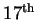century, Newton used both theories to cover the different aspects of light , explaining its periodicy and interference as wave, and it's linear propagation as particle phenomenon. Later, the wave-theory of light has been generally accepted, as scientists like Young and Fresnel could explain most particulate behavior within the realm of the wave-formalism. Except, that is, for one fundamental requirement: The obvious lack of a physical medium which lead to the somewhat far-fetched and unsatisfying Ether'' hypothesis.

On the particle front, Dalton's Law of Multiple Proportions suggested, that chemical substances consist of atoms of different masses. In the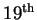century, Boltzmann developed his gas-theory based on atomistic concepts and experiments with cathode rays showed that the electric charges always come in multiples of the elementary charge e which is about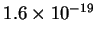Coulomb.

## Plank's Constant

In the year 1900, Max Plank explained the energy spectrum of black body radiation with the ad-hoc assumption, that the possible energy states are restricted to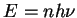, where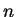is an integer,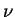the frequency and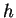the Plank constant, the fundamental constant of quantum physics, with a value of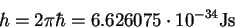In 1888, Hertz demonstrated, that a negatively charged plate would discharge, if exposed to ultraviolet light. Lenard later discovered the kinetic energy of the electrons is independent of the light's intensity but correlated to its frequency, such thatwith some material dependent constant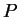. In 1905 Einstein reformulated this relation to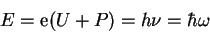interpreting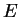as the energy of a light particle, later called a photon.

## Bohr's Atom Model

By analyzing the visible spectrum of Hydrogen, it was found that the light intensity shows very distinct peaks at certain wavelengths. In 1885, Balmer showed that the wavelength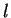is very accurately given by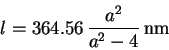(1.1)

This can be generalized to the Rydberg equation, which also accounts for the non-visible parts of the spectrum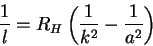(1.2)

This suggests, that the electron in the Hydrogen atom is confined to certain energy levels, which is in contradiction with classical mechanics.

The Bohr-Sommerfeld model accounted for this by introducing a quantum condition: While the electrons are still assumed to circulate the nucleus on their classical orbits, their angular momentum has to be a multiple of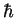. This restriction could be justified by attributing wave properties to the electron and demanding that their corresponding wave functions form a standing wave; however this kind of hybrid theory remained unsatisfactory.

A complete solution for the problem came in 1923 from Heisenberg who used a matrices-based formalism. In 1925, Schrödinger published an alternative solution using complex wave functions. It took two years until Dirac showed that both formalisms were in fact equivalent.

## Wave-Particle Dualism

In 1924, de Broglie assumed that -- in analogy to photons -- every particle of energyand momentum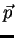can in fact be treated as a wave, whose frequency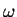and wave-vector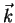are given by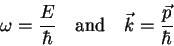(1.3)

This relation was verified in 1927 in diffraction experiments with electrons by Davison and Germer. The inverse effect -- particle behavior of photons -- has been demonstrated 1933 in electron-photon dispersion experiments by Compton.Next: Wave Mechanics Up: Quantum Physics in a Previous: Quantum Physics in a   Contents

(c) Bernhard Ömer - oemer@tph.tuwien.ac.at - http://tph.tuwien.ac.at/~oemer/